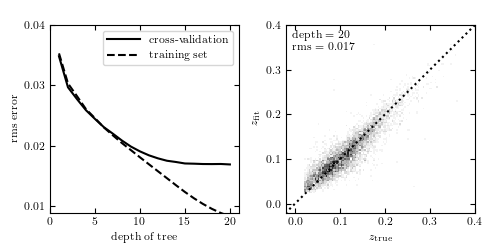# Photometric Redshifts by Random Forests¶

Figure 9.15

Photometric redshift estimation using random forest regression, with ten random trees. Comparison to figure 9.14 shows that random forests correct for the overfitting evident in very deep decision trees. Here the optimal depth is 20 or above, and a much better cross-validation error is achieved.@pickle_results: computing results and saving to 'photoz_forest.pkl'

# Author: Jake VanderPlas
#   The figure produced by this code is published in the textbook
#   "Statistics, Data Mining, and Machine Learning in Astronomy" (2013)
#   To report a bug or issue, use the following forum:
import numpy as np
from matplotlib import pyplot as plt

from sklearn.ensemble import RandomForestRegressor
from astroML.datasets import fetch_sdss_specgals
from astroML.utils.decorators import pickle_results

#----------------------------------------------------------------------
# This function adjusts matplotlib settings for a uniform feel in the textbook.
# Note that with usetex=True, fonts are rendered with LaTeX.  This may
# result in an error if LaTeX is not installed on your system.  In that case,
# you can set usetex to False.
if "setup_text_plots" not in globals():
from astroML.plotting import setup_text_plots
setup_text_plots(fontsize=8, usetex=True)

#------------------------------------------------------------
# Fetch and prepare the data
data = fetch_sdss_specgals()

# put magnitudes in a matrix
mag = np.vstack([data['modelMag_%s' % f] for f in 'ugriz']).T
z = data['z']

# train on ~60,000 points
mag_train = mag[::10]
z_train = z[::10]

# test on ~6,000 distinct points
mag_test = mag[1::100]
z_test = z[1::100]

#------------------------------------------------------------
# Compute the results
#  This is a long computation, so we'll save the results to a pickle.
@pickle_results('photoz_forest.pkl')
def compute_photoz_forest(depth):
rms_test = np.zeros(len(depth))
rms_train = np.zeros(len(depth))
i_best = 0
z_fit_best = None

for i, d in enumerate(depth):
clf = RandomForestRegressor(n_estimators=10,
max_depth=d, random_state=0)
clf.fit(mag_train, z_train)

z_fit_train = clf.predict(mag_train)
z_fit = clf.predict(mag_test)
rms_train[i] = np.mean(np.sqrt((z_fit_train - z_train) ** 2))
rms_test[i] = np.mean(np.sqrt((z_fit - z_test) ** 2))

if rms_test[i] <= rms_test[i_best]:
i_best = i
z_fit_best = z_fit

return rms_test, rms_train, i_best, z_fit_best

depth = np.arange(1, 21)
rms_test, rms_train, i_best, z_fit_best = compute_photoz_forest(depth)
best_depth = depth[i_best]

#------------------------------------------------------------
# Plot the results
fig = plt.figure(figsize=(5, 2.5))
left=0.1, right=0.95,
bottom=0.15, top=0.9)

# left panel: plot cross-validation results
ax.plot(depth, rms_test, '-k', label='cross-validation')
ax.plot(depth, rms_train, '--k', label='training set')
ax.legend(loc=1)

ax.set_xlabel('depth of tree')
ax.set_ylabel('rms error')

ax.set_xlim(0, 21)
ax.set_ylim(0.009,  0.04)
ax.yaxis.set_major_locator(plt.MultipleLocator(0.01))

# right panel: plot best fit

edges = np.linspace(z_test.min(), z_test.max(), 101)
H, zs_bins, zp_bins = np.histogram2d(z_test, z_fit_best, bins=edges)
ax.imshow(H.T, origin='lower', interpolation='nearest', aspect='auto',
extent=[zs_bins, zs_bins[-1], zs_bins, zs_bins[-1]],
cmap=plt.cm.binary)

ax.plot([-0.1, 0.4], [-0.1, 0.4], ':k')
ax.text(0.03, 0.97, "depth = %i\nrms = %.3f" % (best_depth, rms_test[i_best]),
ha='left', va='top', transform=ax.transAxes)

ax.set_xlabel(r'$z_{\rm true}$')
ax.set_ylabel(r'$z_{\rm fit}$')

ax.set_xlim(-0.02, 0.4001)
ax.set_ylim(-0.02, 0.4001)
ax.xaxis.set_major_locator(plt.MultipleLocator(0.1))
ax.yaxis.set_major_locator(plt.MultipleLocator(0.1))

plt.show()